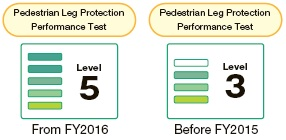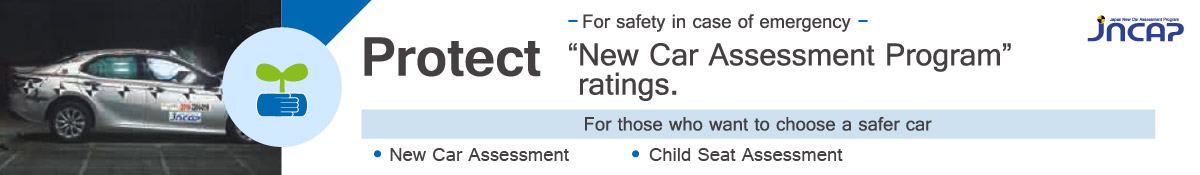# Pedestrian leg Protection Performance Tests

## Testing Methods

In this test, a “leg impactor” (FLEX-PLI) dummy made to represent the leg of an adult male is launched by the testing machine at a speed of 40 km/h at the bumper of the test car. Injuries to the knee and shin area at the point of impact are measured and evaluated on a four-point scale for the degree of injury when the vehicle collides with a pedestrian. This test is applicable for cars for which the height up to the lower edge of the bumper is less than 425 mm. (The test may be conducted even if the height up to the lower edge of the bumper is 425 mm or greater.)
The test area of the bumper that is evaluated in the leg impactor launch test comprises six segments between the bumper (excluding the sides), and the number of locations at which leg injury is measured will vary from 2 to 6 depending on the test vehicle (In the event that there are locations even outside this area that are thought to pose a danger, a test is conducted for these areas).
In the results of evaluation, a higher value indicates better pedestrian leg protection performance.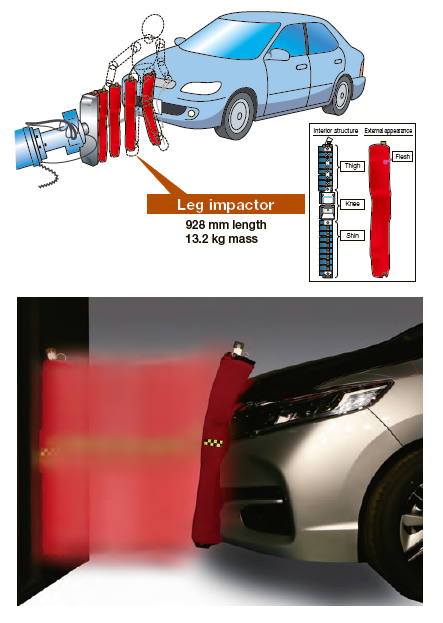## Evaluation Method

The bumper area (excluding the sides) is the basic test area and this area is divided crosswise into three sections numbered Area L1, Area L2 and Area L3 (from right to left).
Each of these three areas is further subdivided into two.
Starting from the right the subdivided areas are labeled as L1A, L1B, L2A, L2B, L3A and L3B.
One or two points in each of the three areas (L1, L2 and L3) that are thought to have the highest injury criteria are selected (although they must be in different sections) and with the injury criteria of the knee and shin obtained from the test as representative criteria, as core is calculated using the evaluation function (sliding scale).
The points are weighted for both the knee and shin areas and the score for each area is calculated. Each area is averaged and the total average score is given as an evaluation.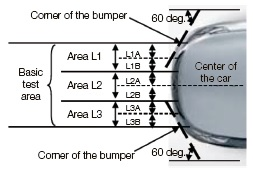Based on the measurements from the sensors attached to the leg impactor, the tibia bending moment and elongation of the knee area medial collateral ligament (MCL), anterior cruciate ligament (ACL) and posterior cruciate ligament (PCL) are calculated using the evaluation function (sliding rule). (As there is Level 2 was above two points and less than 2.67 points. Level 3 was above 2.67 and less than 3.33 points.
Level 4 was above 3.33 points and less than 4.00 points. no risk curve injury criteria for the ACL and PCL, a score of 0, shown as red, is given if the value is 13.0 mm or greater, and the score is represented by the MCL score if the value is less than 13.0 mm.)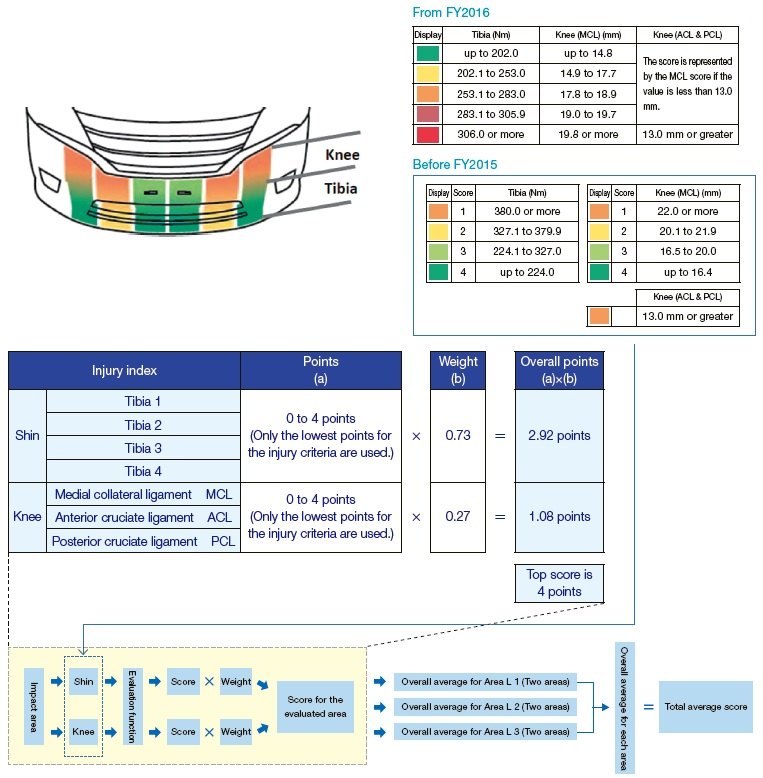## Interpretation of evaluation results

The overall average score is evaluated on a five level rating and to accurately differentiate between the evaluation of different vehicles, the current standard is taken into consideration with two points out of a top score of four being rated as Level 1, while above that is divided into four levels. Level 2 is above two points and less than 2.50 points. Level 3 is above 2.50 and less than 3.00 points. Level 4 is above 3.00 points and less than 3.50 points. Level 5 is above 3.50 points.
Before FY2015, the overall average score was evaluated on a four level rating with two points out of a top score of four being rated as Level 1, while above that was divided into three levels. Level 2 was above two points and less than 2.67 points. Level 3 was above 2.67 and less than 3.33 points. Level 4 was above 3.33 points and less than 4.00 points.